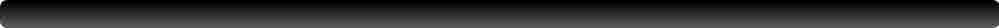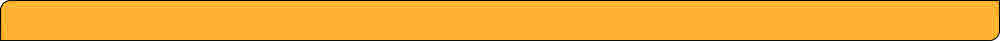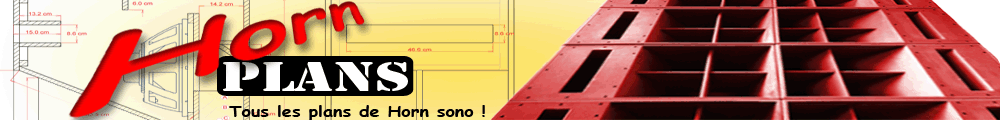﻿ Android CalcAndroid Calc :

Dozens of useful audio calculator:

- Intelligent Limiter Threshold calc

- Eq tools Q<=>BW, Q Proportionnal <=> Constant, etc

- Delay <=> Distance

- Amp gain calc, sensitivity to gain

- Amp volts power calc (watts, volts, RMS, PEAK, etc)

- Electrica formulas calc (find watts, volts, resistance, current)

- dBu/dBV <=> Volts

- SPL from amp gain and distance

- Power ratio to dB

- Average multiples dB values

- Sum multiples dB values

- Sum dB with phase

- Delay <=> Phase <=> Frequency

- Wavelegnth <=> Frequency

- BPM <=> Delay

- Equivalent voltage 1w/1m

- Max speaker cable length

- Max line cable length

- Air attenuation

- Ponderation attenuation

- Speed of sound from temperature, Hygrometry, Pressure

- Crest factor

- DI Index from directivity